2018/07/11

# 序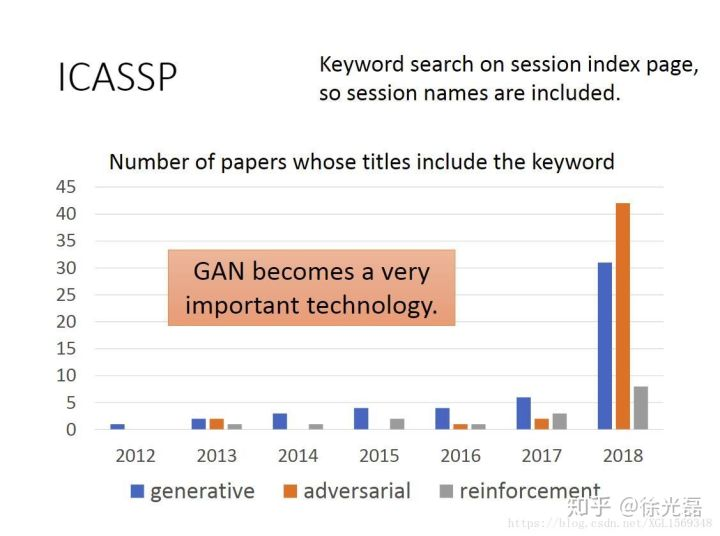GAN的热度可见一番。本文的主要骨干来自台湾大学李宏毅老师的GAN课程以及论文的阅读记录。

# GAN的基础思想

GAN是一类生成模型，和VAE, pixelCNN等生成模型不同的是，GAN引入了NN的对抗环节，来帮助NN生成拟合效果更好的数据。对抗的环节为一个Discriminator NN与一个Generator NN。G的目的为生成拟合效果更好更逼真的数据来骗过D，D的目的为分辨出真实的原始数据和G生成的假数据。如原Paper中打的比方，G是制造假钱的坏人，D是警察专门分辨假钱（“充满暴力与犯罪的例子”）。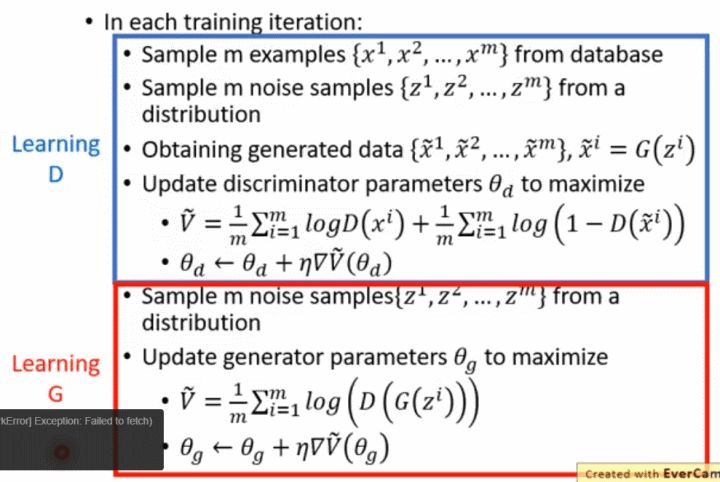如上图，在每一次迭代的过程中，对真实数据采样出x，从某个特定分布（如高斯分布）0中采样z。z作为G的输入，得到一批的生成样本。然后先后梯度更新D和G。

$\theta^{*} = \arg\max \limits_{\theta} \prod^{m} \limits_{i=1} P_{G}(X^{i};\theta)$ $= \arg\max \limits_{\theta} \log \prod^{m} \limits_{i=1} P_{G}(X^{i};\theta)$ $= \arg\max \limits_{\theta} \sum^{m} \limits_{i=1} \log P_{G}(X^{i};\theta)$ $= \arg \max \limits_{\theta} E_{x \sim P_{data}}[\log P_{G}(X^{i};\theta)]$ $= \arg \max \limits_{\theta} \begin{equation*} \int P_{data} \log P_{G}(X;\theta) dx \end{equation*} -$ $\begin{equation*} \int P_{data} \log P_{G}(X) dx \end{equation*}$ $= \arg \min \limits_{\theta} KL(P_{data}||P_{G})$

（注意这里的$X^{i}$是从原始数据集中取样的。) 则得到G的目标就是最小化两者的KL散度。

# fGAN

t是D输出的scalar，我们写作$D(x)$，得到 $D_{f}(P||Q) \approx \max \limits_{D}$ $\begin{equation*} \int_{x} p(x)D(x)dx \end{equation*}$ $-\begin{equation*} \int_{x} q(x)f^{*}(D(x))dx = \max \limits_{D} (E_{x \sim P_{data}}[D(X)] - E_{x \sim P_{G}}[f^*(D(X))] ) \end{equation*}$

# Least Square GAN

Less Square GAN是GAN的一个优化版本。LSGAN解决的问题是，原始D是一个sigmoid二分类器，在D分类效果过好（两个分布解决0或1）的时候，G在跟着D指路优化自身的时候获得的梯度过小，没有办法让两者的分布靠近。在原始的GAN架构中，提出的是减少D训练的次数，防止D分类能力过强，这就对超参的调节提出了很大的要求。

LSGAN将分类的sigmoid输出换成了线性输出，把输出转换成一个回归问题，训练的时候仍然是正样本接近1，负样本接近0。以此优化梯度传递的效果。

# Wasserstein GAN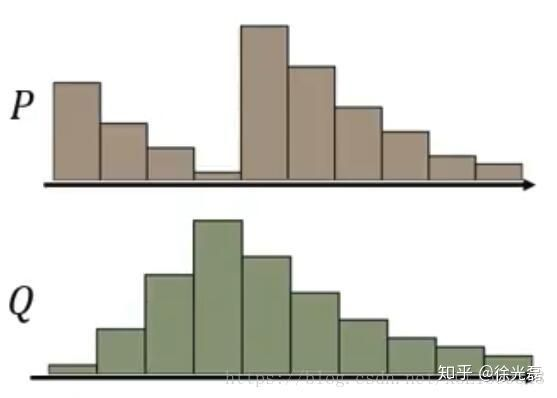（注意这里没有了原来的log，WGAN的D关注指标成为了一个scalar）

1-Lipschitz是符合lipschitz连续条件的一种，这是一个限制函数梯度的条件，一个比通常连续更强的光滑性条件，定义如下 $||f(x_1) - f(x_2)|| \leq K||x_1 - x_2||$ 这里的K常数取1就是1-Lipschitz条件。

（再从直观的角度想一下，假如我们在原始GAN模型中，对D训练次数过多，就会导致正负样本被准确分类，D的曲线自然也就成为一个一端很高，一端很低，其他地方都是0的东西，自然也是不满足Lipschitz条件。）

WGAN的距离函数的思路被后来绝大部分GAN模型借鉴，减少了超参调节的压力，解决了由于JS梯度导致的梯度消失问题。 Wasserstein GAN

# WGAN-GP

WGAN-GP是WGAN的又一次强化版，在WGAN中，我们使用的Weight Clipping方式并不能帮助我们真正实现1-Lipschitz，甚至说逼近效果也不好。

WGAN-GP提出一个加正则项的方法来逼近1-Lipschitz，理想情况下，我们去对D函数每个地方都求导，拿到一个正则项 $max(0,||\nabla_{x}D(x)-1||)$

Improved Training of Wasserstein GANs

（PS：其实$P_{data}$向$P_{G}$的逼近过程中，显然不一定是走直线的。也就有后续的文章把$P_{penalty}$设置为$P_{data}$，证实结果更好。

（ICLR 2018有一个针对完全符合1-Lipschitz的工作：Spectral Normalization for Generative Adversarial Networks，相关解析见：深度学习中的Lipschitz约束：泛化与生成模型 by 苏剑林

# Energy-based GAN

EBGAN把Discriminator修改成了一个Autoencoder，把Discriminator输出的scalar设置为Autoencoder的reconstrcution error（MSE来算距离，这在文中称为能量函数）。基于的假设是如果待评判的图片质量不好，那么重构的结果也不好。

EBGAN对$L_{G}$增加了一个正则项repelling regularizer，来保证使用Autoencoder作为D的时候，不会发生mode collapse的情况。

$S \in R^{s \times N}$，代表从encoder output layer取出的一批样本，$f_{PT}(S)$计算这批样本内的平均余弦距离，以此作为惩罚。

# Loss-sensitive GAN

LSGAN的主要贡献是在D输出scalar的前提下，我们想让D学会一个loss。

Loss-Sensitive Generative Adversarial Networks on Lipschitz Densities

# Boundary Equilibrium GAN

BEGAN的直接目标是优化分布的分布误差之间的相似度，而不是分布的相似度。

$\gamma$是P和Q的任一组合分布，然后用Jensen不等式得出

$m$是分布的期望。

BEGAN的一大优势就是网络结构简单（就是非常单纯地上采样上采样，下采样下采样），降低了训练时对各种trick的需求，什么relu，minibatch，BN全都不需要，而且图像质量被推到了一个很高的水平。

BEGAN: Boundary Equilibrium Generative Adversarial Networks

# Conditional GAN

CGAN这 GAN模型主要的想法是为GAN的生成过程中添加一个标签Y有监督地作为输出的一个监督参考。我们使D同时接受G的输出和标签Y两个变量，除了要判断G的输出是否realistic，同时要判断输出与Y 是否有关联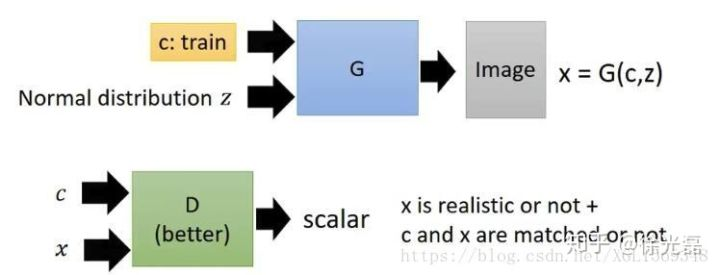# Cycle GAN

Cycle GAN想要解决的问题是无监督情况下地去实现Conditional GAN的目标。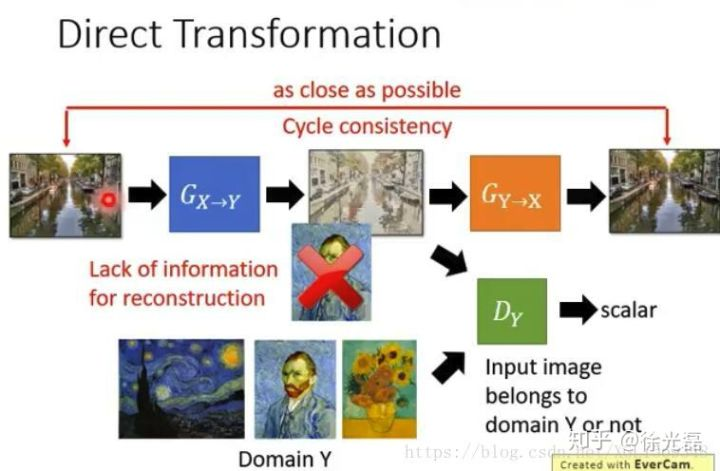Cycle的做法也可以是走一个双向的路径，既然我们训练了$G_{Y->X}$，那么我们完全可以开一条类似的循环生成路径来把目标domain的图片转化为原始domain的图片（如下图）。

$L_{cyc}(G,F)=E_{x \sim p_{data}(x)}[||F(G(x)-x)||]+E_{y \sim p_{data}(y)}[||G(F(y)-y)||]$ 这里的$G(x)$，$F(y)$分别对应前文的$G_{X->Y}$，$G_{Y->X}$。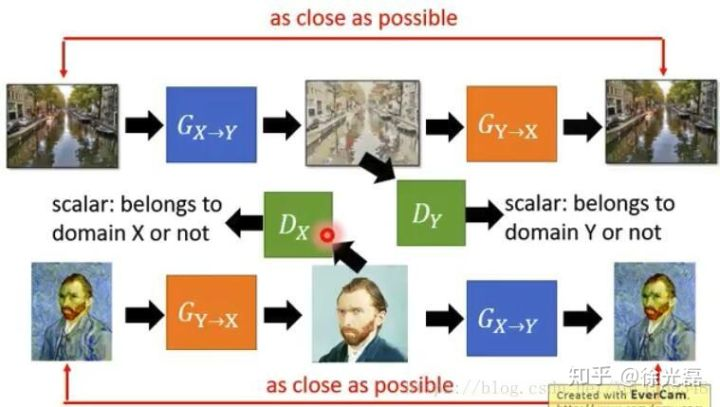Unpaired Image-to-Image Translation using Cycle-Consistent Adversarial Networks

（PS：Cycle GAN这样的方法只能说大部分时候是work的，但是毛病也不少，举例NIPS2017的CycleGAN: a Master of Steganography指出Cycle GAN的做法，不能保证output图片中可用于重构input的信息是以原始方式保存的。

# Star GAN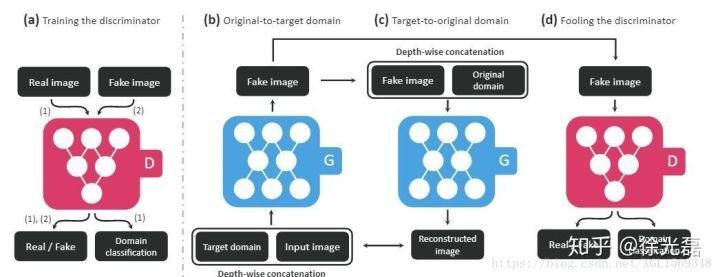StarGAN: Unified Generative Adversarial Networks for Multi-Domain Image-to-Image Translation

# CoGAN

CoGAN想要解决的问题，也是Unsupervised Conditional GAN。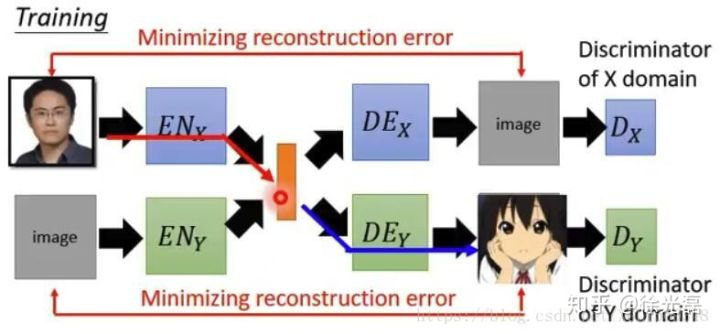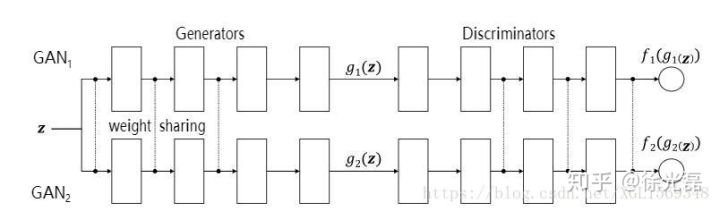Coupled Generative Adversarial Networks